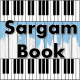# Burn (Ellie Goulding) Sargam Notes

Song Burn From Album  Halcyon (2012) Sargam And Harmonium Notes Now Available On Sargam Book With Video Harmonium Tutorial. Burn Song  Sung By Ellie Goulding.

Song : Burn
Album : Halcyon (2012)
Singer : Ellie Goulding
Western Notes : Website 1, Website 2, Website 3
Classical Sargam Notes: www.sargambook.com

We, we don’t have to worry ’bout nothing
Ma, Ga(k) Ga(k) Ga(k) Ga(k) Ga(k)
Ga(k) Ga(k) Re(k) ni(k)

‘Cause we got the fire, and we’re burning one hell of a something
Re(k) Re(k) Ga(k) Re(k) Ma,
Ga(k) Ga(k) Ga(k) Ga(k) Ga(k)
Ga(k) Ga(k) Re(k) ni(k)
They, they gonna see us from outer space, outer space
Ma, Ga(k) Ga(k) Ga(k) Ga(k) Ga(k)
Ga(k) Ga(k) Re(k) ni(k)
Ga(k) Ma Re(k)
Light it up, like we’re the stars of the human race, human race
Ga(k) Re(k) Ma,
Ga(k) Ga(k) Ga(k) Ga(k) Ga(k)
Ga(k) Ga(k) Re(k) ni(k)
Ga(k) Ma Re(k)
When the light started out they don’t know what they heard
Ni(k) SA RE(k) RE(k) SA Ni(k)
Dha(k) Ma(t) Dha(k)
Ma(t) Ma Ga(k)
Strike the match, play it loud, giving love to the world
Ni(k) SA RE(k) RE(k) SA Ni(k)
Dha(k) Ma(t) Dha(k)
Ma(t) Ma Ga(k)
We’ll be raising our hands, shining up to the sky
Ni(k) SA RE(k) RE(k) SA Ni(k)
Dha(k) Ma(t) Dha(k)
Ma(t) Ma Ga(k)
‘Cause we got the fire, fire, fire
Ga(k) Ma Ga(k) Re(k)
Re(k), Re(k), Re(k)
Yeah we got the fire, fire, fire
Ga(k) Ma Ga(k) Re(k)
Re(k), Re(k), Re(k)

And we gonna let it burn, burn, burn, burn
Ga(k) Ga(k) Ga(k) Ma Ga(k) Re(k) Re(k),
Re(k), Re(k), Re(k)
We gonna let it burn, burn, burn, burn
Ga(k) Ga(k) Ga(k) Ma Ga(k) Re(k) Re(k),
Re(k), Re(k), Re(k) Ga(k)
Gonna let it burn, burn, burn, burn
Ga(k) Ga(k) Ga(k) Ma Ga(k) Re(k) Re(k),
Re(k), Re(k), Re(k)
We gonna let it burn, burn, burn, burn
Ga(k) Ga(k) Ga(k) Ma Ga(k) Re(k) Re(k),
Re(k), Re(k), Re(k)